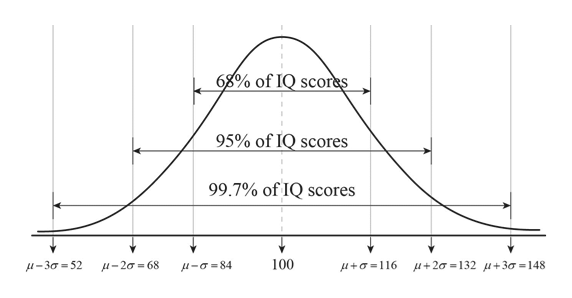68% of IQ scor95% of 10) scores99.7% of IQ scoresμ-3σ = 52μ-2σ = 68μ-σ=84100μ+σ=116μ+2σ = 132μ+3σ = 148

Question

Based on the model, what percent of people should have IQ scores above 116? What percent of people should have IQ scores between 68 and 84? What percent of people should have IQ scores above 132?help_outlineImage Transcriptionclose68% of IQ scor 95% of 10) scores 99.7% of IQ scores μ-3σ = 52 μ-2σ = 68 μ-σ=84 100 μ+σ=116 μ+2σ = 132 μ+3σ = 148 fullscreen
Step 1

Given a normal distribution with  mean 100 and standard deviation 16.

IQ score 116 is one standard deviation (16)away  from mean 100. From given diagram of empirical rule we know that with in one standard deviation of mean the data is 68%. So from 84 to 116 percentage of data is 68%. As this is symmetric and normal curve the mean divides the region from 84 to 116 equally. So the region from 100 to 116 will have half of 68% that is 34%.

The percentage of people with IQ score from 100 to 116 is 34%

THe percentage of people with  more than 100 IQ score is 50% as 100 is the mean.

So the percentage of data with IQ score above 116 = percentage of people with I score greater than 100 - percentage of people with IQ score between 100 and 116.

the percentage of data with IQ score above 116 = 50%-34% = 16%

Step 2

The percentage of people with IQ score  from 84 to 100 = 34%.

The percentage of people with IQ score 68 to 132 is 95% and as 100 is mean the percentage of people with IQ score from 68 to 100 is 47.5%.

The percentage of people wi...

Want to see the full answer?

See Solution

Want to see this answer and more?

Our solutions are written by experts, many with advanced degrees, and available 24/7

See Solution
Tagged in

Statistics